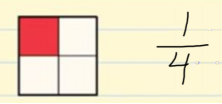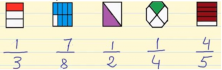Smartick is an online platform for children to master math in only 15 minutes a day

Jul15

# Understand What a Fraction Is and When It Is Used

With this post, we are going to understand the notion of a fraction as a part of a whole, as a division of natural numbers and its position on the number line.

### What is a fraction?

A fraction represents the number of parts that we have of a whole that is divided into equal parts. Fractions are represented by two numbers that are separated by a fraction line.### Fraction Terms

The terms that we use for fractions are the “numerator” and the “denominator.” The numerator is the number of parts that we have and the denominator is the total number of parts that make up the whole.

Let’s look at an example:

We have different shapes and we’ll divide each one of them into a number of equal parts, which is the denominator.

The number of colored parts is the numerator.### How do we read a fraction?

The numerator is read with cardinal numbers: one, two, three, ten, twenty-four,…

The denominator is read with fractional numbers: halves, thirds, fourths,…

### Types of Fractions

• Proper Fractions: When the numerator is less than the denominator.
• Improper Fractions: When the numerator is greater than the denominator.
• Whole Fractions: When the numerator is equal to the denominator.

Check out previous posts from our blog where you can learn much more about fractions:

And if you want to learn much more elementary math, try Smartick for free and have fun!